Courses

Matrix-Match & Integer Answer Type Questions: Waves | JEE Advanced Notes | Study Physics 35 Years JEE Main & Advanced Past year Papers - JEE

JEE: Matrix-Match & Integer Answer Type Questions: Waves | JEE Advanced Notes | Study Physics 35 Years JEE Main & Advanced Past year Papers - JEE

The document Matrix-Match & Integer Answer Type Questions: Waves | JEE Advanced Notes | Study Physics 35 Years JEE Main & Advanced Past year Papers - JEE is a part of the JEE Course Physics 35 Years JEE Main & Advanced Past year Papers.
All you need of JEE at this link: JEE

Match the Following

DIRECTIONS (Q. No. 1-2) : Each question contains statements given in two columns, which have to be matched. The statements in Column-I are labelled A, B, C and D, while the statements in ColumnII are labelled p, q, r and s. Any given statement in Column-I can have correct matching with ONE OR MORE statement(s) in Column-II. The appropriate bubbles corresponding to the answers to these questions have to be darkened as illustrated in the following example :

If the correct matches are A-p, s and t; B-q and r; C-p and q; and D-s then the correct darkening of bubbles will look like the given.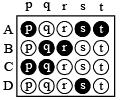Q.1. Each of the properties of sound listed in the column A primarily depends on one of the quantities in column B. Write down the matching pairs from the two columns.        (1980)

 Column A Column B A. pitch p. Waveform B. quality q. fr equency C. loudness r. intensity

Ans. A → q; B → p; C → r

Solution.

 (A) Pitch q. fr equency (B) quality p. waveform (C) loudn ess r. intensity

Q.2. Column I shows four systems, each of the same length L, for producing standing waves. The lowest possible natural frequency of a system is called its fundamental frequency, whose wavelength is denoted as λf. Match each system with statements given in Column II describing the nature and wavelength of the standing waves.   (2011)

Ans. A → p, t; B → p, s; C → q, s; D → q, r

Solution. (A) Pipe closed at one end

Waves produced are longitudinal (sound waves)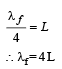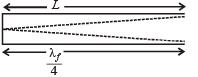(p, t) are correct matching
(B) Pipe open at both ends

waves produced are longitudinal (sound waves)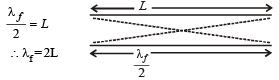(p, s) are correct matching.

(c) Stretched wire clamped at both ends

Waves produced are transverse in nature. (waves on string)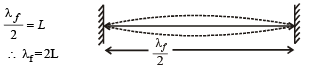(q, s) are correct matching. (D) Stretched wave clamped at both ends & mid point Waves produced are transverse in nature (waves on string)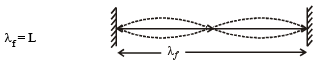(q, r) are correct matching.

Integer Value Correct Type

Q.1. A  20  cm long string, having a mass of 1.0 g, is fixed at both the ends. The tension in the string is 0.5 N. The string is set into vibrations using an external vibrator of frequency 100 Hz. Find the separation (in cm) between the successive nodes on the string.        (2009)

Ans. 5

Solution.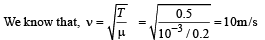The wavelength of the wave established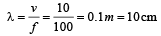∴ The distance between two successive nodes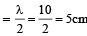Q.2. A stationary source is emitting sound at a fixed frequency f0, which is reflected by two cars approaching the source.

The difference between the frequencies of sound reflected from the cars is 1.2% of f0. What is the difference in the speeds of the cars (in km per hour) to the nearest integer ?

The cars are moving at constant speeds much smaller than the speed of sound which is 330 ms–1.         (2010)

Ans. 7

Solution.

Let v be the speed of sound and vc and f0 the speed and frequency of car.

The frequency of sound reflected by the car is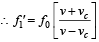Differentiating the above equation w.r.t. vc ,we get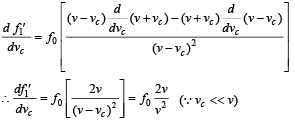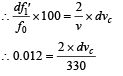∴ dvc = 0.198 m/s ≈ 7 km/h

Q.3. Wh en two progressive waves y1 = 4 sin (2x – 6t) and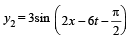are superimposed, the amplitude of the resultant wave is

Ans. 5

Solution.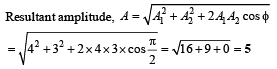Q.4. Four harmonic waves of equal frequencies and equal intensities I0 have phase angles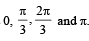When theyare superposed, the intensity of the resulting wave is nI0. The value of n is             (JEE Adv. 2015)

Ans. 3

Solution.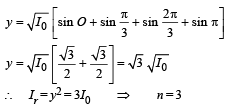The document Matrix-Match & Integer Answer Type Questions: Waves | JEE Advanced Notes | Study Physics 35 Years JEE Main & Advanced Past year Papers - JEE is a part of the JEE Course Physics 35 Years JEE Main & Advanced Past year Papers.
All you need of JEE at this link: JEEUse Code STAYHOME200 and get INR 200 additional OFF Use Coupon Code

Top Courses for JEE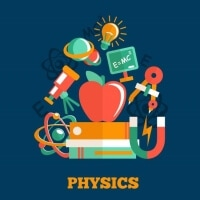Physics 35 Years JEE Main & Advanced Past year Papers

88 docs|49 tests

Top Courses for JEETrack your progress, build streaks, highlight & save important lessons and more!

,

,

,

,

,

,

,

,

,

,

,

,

,

,

,

,

,

,

,

,

,

;